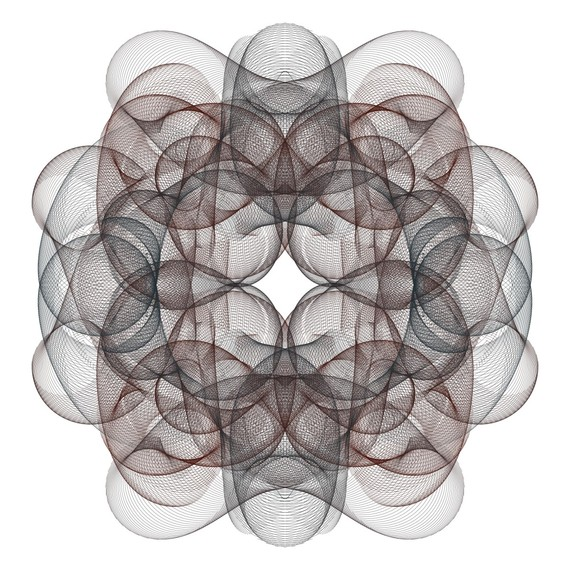# These Beautiful Images Are Created By Drawing Circular Arcs

I have created the following images by thousands of circular arcs which are defined with trigonometric functions. Such families of arcs are very useful to introduce beautiful shapes. At the end of this post you can see the mathematical description of "6,000 Arcs (4)". Also, in my previous posts you can see some images that I made by using line segments, circles, ellipses and squares.

6,000 Arcs (4)This image shows 6,000 circular arcs. For each k=1,2,3,...,6000 the endpoints of the k-th arc are

(S(k)cos(A(k))+X(k), S(k)sin(A(k))+Y(k))

and

(S(k)cos(B(k))+X(k), S(k)sin(B(k))+Y(k)),

and the following point is located on the k-th arc,

(S(k)cos(C(k))+X(k), S(k)sin(C(k))+Y(k))

where

S(k)=(1/20)+(6/15)(sin(32πk/6000))2,

A(k)=(214πk/6000)+(π/20)+(19π/20)(sin(32πk/6000))4,

B(k)=(214πk/6000)-(π/20)-(19π/20)(sin(32πk/6000))4,

C(k)=(214πk/6000),

X(k)=(21/19)cos(18πk/6000)+(21/38)(cos(34πk/6000))3,

Y(k)=(21/19)sin(18πk/6000)+(21/38)(sin(58πk/6000))3.